# Test: Triangles- 1

## 20 Questions MCQ Test Quantitative Aptitude for GMAT | Test: Triangles- 1

Description
Attempt Test: Triangles- 1 | 20 questions in 40 minutes | Mock test for GMAT preparation | Free important questions MCQ to study Quantitative Aptitude for GMAT for GMAT Exam | Download free PDF with solutions
QUESTION: 1

### ABC is a triangle in which AB = 4 cm and BC = 7cm. What is the possible range of values of CA?

Solution:

Possible range of values of CA will be |AB - BC| < CA < |AB + BC|

So possible range of values of CA will be 3 < CA <11

QUESTION: 2

Solution:
QUESTION: 3

###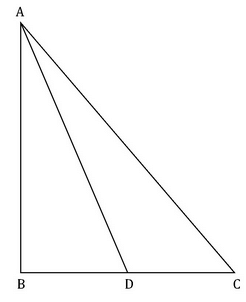In the given figure, AB = 6cm. Find the area of the triangle ADC.    CD = 4cm Angle BAD = 30o and Angle ADC = 120o

Solution:
QUESTION: 4

In the given figure, AE is perpendicular to BC and BD = DC. If the area of triangle ABD = 6cm2, what is the area of triangle ADC?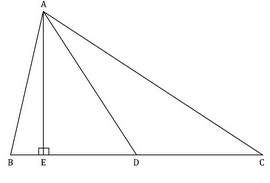Solution:
QUESTION: 5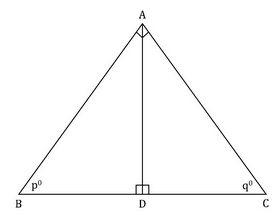From the given diagram, find the area of triangle ABC.

1. po = qo
2. The product of AB and AC is 9.
Solution:
QUESTION: 6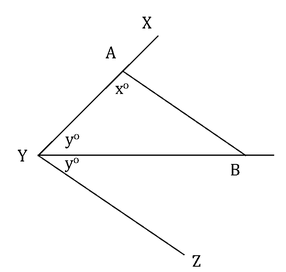If yo = 45o, is xo = 90o?

I.  AB is parallel to YZ

II.  AB = AY

Solution:

Steps 1 & 2: Understand Question and Draw Inferences

In ΔABY,

xo + yo + Angle ABY = 180o

• xo + 45o + Angle ABY = 180o
• xo + Angle ABY = 135o

We need to find if x is a right angle or not, i.e. if x = 90o.

For this we need the value of Angle ABY.

Step 3: Analyze Statement 1

Given that AB is parallel to YZ.

• xo + 2yo = 180 (Since both of them are consecutive interior angles).

Given that y = 45o

• x = 90o

Therefore, statement 1 is sufficient to arrive at a unique answer.

Step 4: Analyze Statement 2

Given AB = AY.

Therefore, Angle ABY = yo = 45o (given)

Therefore, xo + 45o = 135o

• x = 90o

Therefore, statement 2 is sufficient to arrive at a unique answer.

Step 5: Analyze Both Statements Together (if needed)

Since we arrived at a unique answer in at least one of step 3 and step 4, this step is not necessary.

QUESTION: 7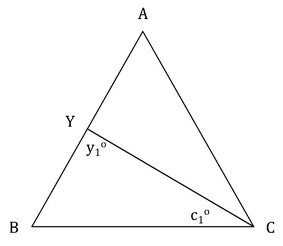In the triangle above, Is AB > AC > BC?

I.  y1 = c1

II.  y1 = 60o

Solution:

Steps 1 & 2: Understand Question and Draw Inferences

ABC is a triangle and CY is a line segment cutting AB at Y.

Angle BYC = y1o and Angle BCY = c1o

We need to find if the statement AB > AC > BC is true or not.

For this we it is enough to get a relation among angles A, B and C since the sides of a triangle follow the same relation as the angles opposite to them.

Step 3: Analyze Statement 1

y1 = c1

Therefore, in ΔBYC,

BY = BC

But AB = BY + YA

• AB > BC

However, we cannot infer any relationship between BC and AC (or AB and AC) from the given information.

(In other words, BC could be greater than AC or less than AC or equal to AC).

Therefore, statement 1 is not sufficient to arrive at a unique answer.

Step 4: Analyze Statement 2

y1 = 60o

Therefore, Angle A + Angle ACY = 60 (Exterior angle = sum of two opposite interior angles).

• Angle A < 60o

This leads us to 3 cases as follows:

Case 1: One of Angle B and Angle C is greater than Angle A in which case one of AB and AC is greater than BC and the other less than BC. But we don’t know which one will be greater or which one will be less than BC.

Case 2: Angle B and Angle C are equal. Hence, using, A+B+C = 180o, we can say that A+2B = 180and A<60o. So, 2B>120o or B>60o. Hence, both B and C are greater than A. So, AC and AB are equal and both are greater than BC.

Case 3: Angle B and Angle C are unequal and both are greater than Angle A in which case both AB and AC are greater than BC but we do not know which one among AB and AC is bigger.

Therefore, BC cannot be the longest side.

Therefore, statement 2 is not sufficient to arrive at a unique answer.

Step 5: Analyze Both Statements Together (if needed)

Combining both the statements, we have:

y1 = c1= 60o and Angle A < 60o

Therefore, Angle B = 60o and Angle C > 60o

Therefore, Angle C > Angle B > Angle A

Therefore, AB > AC > BC

Both the statements together are sufficient to arrive at a unique answer.

QUESTION: 8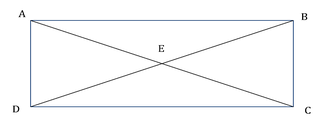In the figure shown above, point E is the intersection point of the diagnoals AC and BD of rectangle ABCD. Is AB greater than BC?

1.  AE = 5 cms and AB = 7.25 cm
2. The perimeter of AEB is greater than the perimeter of BEC
Solution:

Steps 1 & 2: Understand Question and Draw Inferences

In the given rectangle ABCD, we need to find if AB > BC, i.e., if the length is greater than the breadth.

Note that AC and BD are the diagonals of the rectangle and E is their point of intersection.

In right triangle ADC, by Pythagoras Theorem,

AD2 + DC2 = AC2  .  .  . (1)

Similarly, in right triangle DCB,

DC2 + BC2 = DB2  .  .  . (2)

Equation (1)   – Equation (2):

AD2 – BC2 = AC2 – DB2

Being opposite sides of a rectangle, AD = BC

Therefore, the above equation becomes:

0 = AC2 – DB2Therefore, AC = DB.

Thus, we observe that the two diagonals of the rectangle are equal.

As we know that diagonals of a rectangle bisect each other, we can write

AE = BE = CE = DE = AC/2 = BD/2

(Now, it is also easy to notice that the rectangle is symmetrical about point E.

Therefore, point E will divide each diagonal in half. )

Step 3: Analyze Statement 1

Given AE = 5

• AC = 10

Also, given that AB = 7.25

Notice that ABC is a right angled triangle. Applying Pythagoras theorem

AC2 = AB2 + BC2

• BC2 = 102 – 7.252

Therefore, we can calculate the value of BC and so we can arrive at a unique answer on whether AB is greater than BC or not.

Statement 1 is sufficient to arrive at a unique answer.

Step 4: Analyze Statement 2

Perimeter of AEB > Perimeter of BEC

• AE + BE + AB > BE + CE + BC
• AB > BC (Since we already noted that AE = BE = CE = DE)

Statement 2 is sufficient to arrive at a unique answer.

Step 5: Analyze Both Statements Together (if needed)

We arrived at a unique answer in step 3 and step 4 above. Therefore, this step is not needed.

QUESTION: 9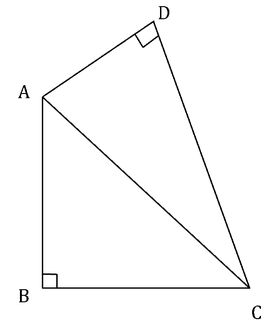I.  Angle CAB = Angle BCA

II.  CD > AB

Solution:

Steps 1 & 2: Understand Question and Draw Inferences

Given a quadrilateral ABCD with right angles at B and D.

We need to find if AD > BC is true.

Step 3: Analyze Statement 1

Given that Angle CAB = Angle BCA

Therefore, in ΔABC,

Angle CAB = Angle BCA = 45o

Therefore, BC = AB

This doesn’t give us any relation between AD and BC.

So statement 1 is not sufficient to arrive at a unique answer.

Step 4: Analyze Statement 2

Given that CD > AB.

In the right angled triangle ABC, we have

AC2 = AB2 + BC2

Similarly, in the right angled triangle ADC, we have

Therefore,

AD2 + CD2 = AB2 + BC2……………. (I)

Given that CD > AB

• CD2 > AB2

In other words, a term in the LHS of (I) is greater than another term in the RHS of (I)

So for (I) to be true (the equality to hold true), the remaining terms on either sides of LHS and RHS should compensate for the imbalance created by CD2 and AB2.

From (I),

Let’s say CD2 – AB2 = k (a positive number since CD2 > AB2)

Then

BC2 – AD2 = k (the same positive number).

Therefore, statement 2 is sufficient to arrive at a unique answer.

Step 5: Analyze Both Statements Together (if needed)

We arrived at a unique answer in step 4 above. Therefore, this step is not needed.

QUESTION: 10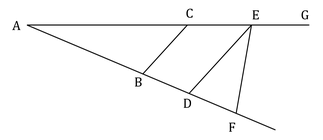If BC || DE, then is AC > BC?

(1) DE = EF

Solution:

Steps 1 & 2: Understand Question and Draw Inferences

We have to determine if AC > BC

In triangle ABC,

Let Angle BAC = a?

Angle ABC = x?

And, Angle ACB = y?

We know that in a triangle, the side opposite to the bigger angle is longer.

Therefore, AC > BC if x > a

So, the question essentially becomes to determine if x > a

We are given that BC || DE

Since corresponding angles are equal,

And, Angle DEA = y?

In the light of this information and the information given in Statements 1 and 2, we need to determine if x > a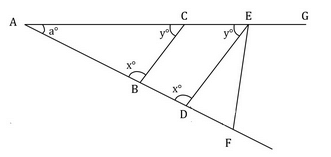Step 3: Analyze Statement 1

Given DE = EF

We know that in a triangle, the angles opposite to equal sides are equal.

Therefore, in Triangle DEF,

Angle EDF = Angle DFE

Now, Angle EDF = (180 – x)?     (Since Angles EDF and ADE are angles on a straight line, their sum equals 180?)

Therefore, Angle EDF = Angle DFE = (180 – x)?

In triangle AFE,

Angle GEF is the exterior angle.

By Exterior Angle Theorem, the exterior angle is equal to the sum of the interior opposite angles.

Angle GEF = Angle EAF + Angle AFE.

Let’s assume angle GEF = z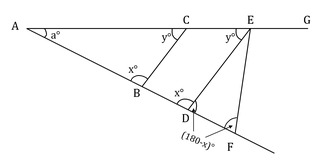z = a° + (180-x)°

Rearranging the terms, we get:

x° ­- a° = 180° - z

Since, z is the exterior angle of a triangle, it can never be greater than 180°

Thus, x – a > 0

Or, x > a

Thus, Statement 1 alone is sufficient to determine if x  > a

Step 4: Analyze Statement 2

This implies, x° > y°

However, this doesn’t give us any clue about whether x > a

For example, using only Statement 2, both these sets of (x°, y°, a°) are possible:

(50°, 40°¸90°) and (90°, 30°, 60°)

In the first case, x < a and in the second case, x > a

Thus, Statement 2 alone is not sufficient to determine if x > a

Step 5: Analyze Both Statements Together (if needed)

Since we arrived at a unique answer in step 3 above, this step is not needed.

QUESTION: 11

A right angled triangle, right angled at point B has angles such that a<c<b where a, b, c correspond to the angles at points A, B, C. If the area of the triangle is 2 which of the following inequality indicates the range of values of side BC.

Solution:

Since it is given that ΔABC is right angled at B, Angle ABC = 90

Also, area of ΔABC = ½ * AB * BC = 2

AB*BC = 4

But it is given that a < c

Therefore, BC < AB

In the extreme case of BC = AB (which is not possible according to the given condition),

BC = AB = 2

However, since BC < AB

BC should be less than 2 and AB should be greater than 2.

Therefore, BC < 2

Also, since BC is a side of a triangle, it is positive and greater than zero

Therefore, BC > 0

Combining the above two inequalities, we get:

0 < BC < 2

QUESTION: 12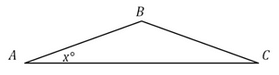In the given isosceles triangle, if AB = BC = 2 and x = 22.5°, what is the area of the triangle?

Solution:

Step 1: Question statement and Inferences

You know that the equal sides are 2 and the equal angles are 22.5°.

To find the area, you need the base and height.

Step 2: Finding required values

Rotate the triangle so that CB is the base, and extend the line.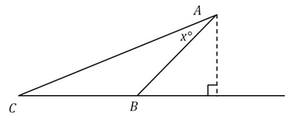Knowing x is 22.5°, the opposite angle is 22.5°, and angle ABC is 135°. This makes the new dotted triangle a 45°-45°-90° triangle with a hypotenuse of 2.

Step 3: Calculating the final answer

Knowing the hypotenuse of the 45o-45o-90o triangle has a length of 2, call the other two sides y, and use the Pythagorean Theorem to find y:

y2+y2=2

2y2=4

y2=2

y=√2

So the dotted line, which is the height of our target triangle, is √2. The base is 2, so base × height / 2 is √2.

QUESTION: 13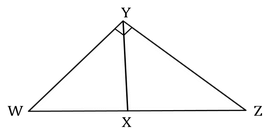In the triangle above, what is the measure of Angle YZX?

(1)         Angle YWX = angle WYX = 30°

(2)         Triangles WXY and XYZ are isosceles triangles

Solution:

Steps 1 & 2: Understand Question and Draw Inferences

We are given that Angle WYZ = 90°

In triangle WYZ, by applying Angle Sum Property, we get:

Angle ZWY + Angle WYZ + Angle YZW = 180°

Angle ZWY + 90° + Angle YZW = 180°

Angle ZWY + Angle YZW = 90°  -  - -(1)

We need to find the value of Angle YZW (please note that this angle can also be labelled as Angle YZX)

Step 3: Analyze Statement 1

Angle YWX = angle WYX = 30°

Applying this information in Equation 1, we get:

30° + Angle YZW = 90°

Angle YZW = 60°

Thus, Statement (1) is sufficient to determine a unique value of Angle YZW.

Step 4: Analyze Statement 2

Triangles WXY and XYZ are isosceles triangles

We don’t know which sides of triangle XYZ are equal.

One possibility is that ZX = XY, and therefore, Angle XYZ = Angle YZX

• So, possible values of (Angle YZX, Angle XYZ, Angle ZXY) can be (40°, 40°, 100°), (30°, 30°, 120°) etc.

Another possibility is that ZX = ZY, and therefore Angle XYZ = Angle ZXY

• So, possible values of (Angle YZX, Angle XYZ, Angle ZXY) can be (100°, 40°, 40°), (120°, 30°, 30°) etc.

Thus, multiple values of angle YZX are possible from Statement 2.

Statement (2) is not sufficient.

Step 5: Analyze Both Statements Together (if needed)

You get a unique answer in Step 3, so this step is not required

QUESTION: 14

Is the perimeter of triangle ABC greater than 6 cm?

(1) One side of triangle ABC measures 3 cm

(2) The lengths of the three sides of triangle ABC are consecutive integers

Solution:

Steps 1 & 2: Understand Question and Draw Inferences

Let’s say the sides of the triangle are a, b, and c.

Let the perimeter of the triangle be X.

Thus, X = a + b + c

We need to find whether X > 6

Since we don’t have any other information, let’s move on to the analysis of the statement 1.

Step 3: Analyse Statement 1

Statement 1 says: One side of triangle ABC measures 3 cm.

Let’s assume a = 3 cm

Now, we know that the sum of two sides of a triangle is always greater than the third side.

So, we can write:

b + c > a

Adding a to both sides of this inequality, we get:

a + b + c > 2a

That is, X > 2a

Substituting a = 3 cm in this inequality, we get:

X > 6 cm

Thus, Statement 1 alone is sufficient to answer the question.

Step 4: Analyse Statement 2

Statement 2 says: The lengths of the three sides of triangle ABC are consecutive integers.

So, the lengths of the sides are n, (n+1), (n+2) cm, where n is a positive integer.

Perimeter X = n + (n+1) + (n+2)

X = 3n + 3

X = 3(n+1)

Now, we know that the sum of two sides of a triangle is always greater than the third side.

This means, we can write:

n + (n+1) > n + 2

2n + 1 > n + 2

n > 1

Adding 1 to both sides of this inequality:

n + 1 > 2

We know that multiplying both sides of an inequality with a positive number doesn’t change the inequality. Therefore, multiplying both sides of the above inequality with 3:

3(n+1) > 6

This means, X > 6

Thus, Statement 2 alone is sufficient to determine if X > 6

Step 5: Analyse Both Statements Together (if needed)

Since we got the answer to the question in Steps 3 and 4, we don’t need to perform this step.

QUESTION: 15

In a triangle ABC,∠ A = 900, AL is drawn perpendicular to BC, Then ∠ BAL is equal to: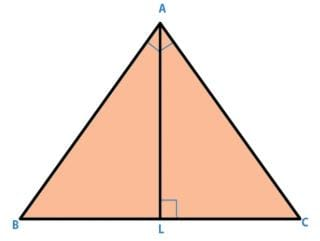Solution:

∠ BAL + ∠ B + 900 = 1800
or, ∠ BAL + ∠ B = 900
or, ∠ BAL = 900 - ∠ B ----------- (1)
Now, in Δ ABC,
∠ ACB + ∠ B + ∠ A = 1800
∠ ACB = 900
-∠ B ----- (2)
From, (1) and (2),
∠ BAL = ∠ ACB.

QUESTION: 16

Consider the triangle shown in the figure where BC = 12 cm, Db = 9 cm, CD = 6 cm and What is the ratio of the perimeter of the triangle ADC to that of the triangle BDC ?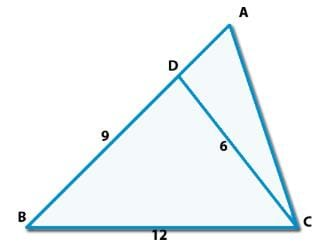Solution:

Here, ∠ACB = c+180-(2c-b) = 180-(b+c)
So, We can say that Δ BCD and &delta ABC will be similar.
According to property of similarity,
AB/12 = 12/9
Hence,
AB = 16
AC/6 = 12/9
AC = 8
Hence, AD = 7 and AC = 8
Now,
Perimeter of Delta; ADC / Perimeter of &delta BDC,
= (6+7+8)/(9+6+12)
= 21/27 = 7/9.

QUESTION: 17

In a triangle ABC, the internal bisector of the angle A meets BC at D. If AB = 4, AC = 3 and ∠  A = 600, then length of AD is :

Solution: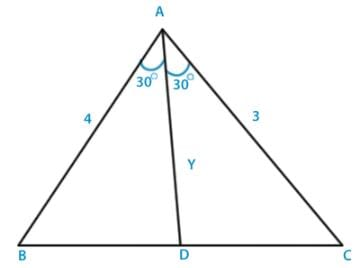Let BC = x and Ad = y, then as per bisector theorem,
BD /DC = AB /AC = 4 /3
Hence, BD = 4x/7 and DC = 3x/7
Now, in Δ ABD using cosine rule,
cos 300 = [{42 +y2 - (16x2/49)} /2*3*y]
Or, 4√3y =[{16 +y2 - (16x2/49)}] --------- (i)
cos 300 = [{32 +y2 - (9x2/49)} /2*3*y]
Or, 3√3y =[{9 +y2 - (9x2/49)}] --------- (ii)
From equation (i) and (ii), we get
y = 12√3 /7.

QUESTION: 18

The point of intersection of the altitudes of a triangle is called its:

Solution:
QUESTION: 19

In Δ PQR, PS is the bisector of ∠ P and PT ⊥ OR, then ∠ TPS is equal to:

Solution:

∠1 + ∠2 = ∠3 [PS is bisector.] ------ (1)
∠Q = 900 - ∠1
∠R = 900 -∠2 - ∠3
So,
∠Q - ∠R = (900 - ∠1) - (900 - ∠2 - ∠3)
∠Q - ∠R = ∠2 + ∠3 - ∠1
∠Q - ∠ R = ∠2 + (∠1 + ∠2) -∠1[using equation 1]
∠Q - ∠R = 2 ∠2
1/2 * (∠Q - ∠R) = ∠TPS.

QUESTION: 20

Two right angled triangles are congruent if :
I.The hypotenuse of one triangle is equal to the hypotenuse of the other.
II.a side for one triangle is equal to the corresponding side of the other.
III.Sides of the triangles are equal.
IV. An angle of the triangle are equal.
Of these statements, the correct ones are combination of:

Solution:

Two right angled triangle are congruent if the hypotenuse of one triangle is equal to the hypotenuse of the other and a side of one triangle is equal to the corresponding side of the other triangleUse Code STAYHOME200 and get INR 200 additional OFF Use Coupon Code